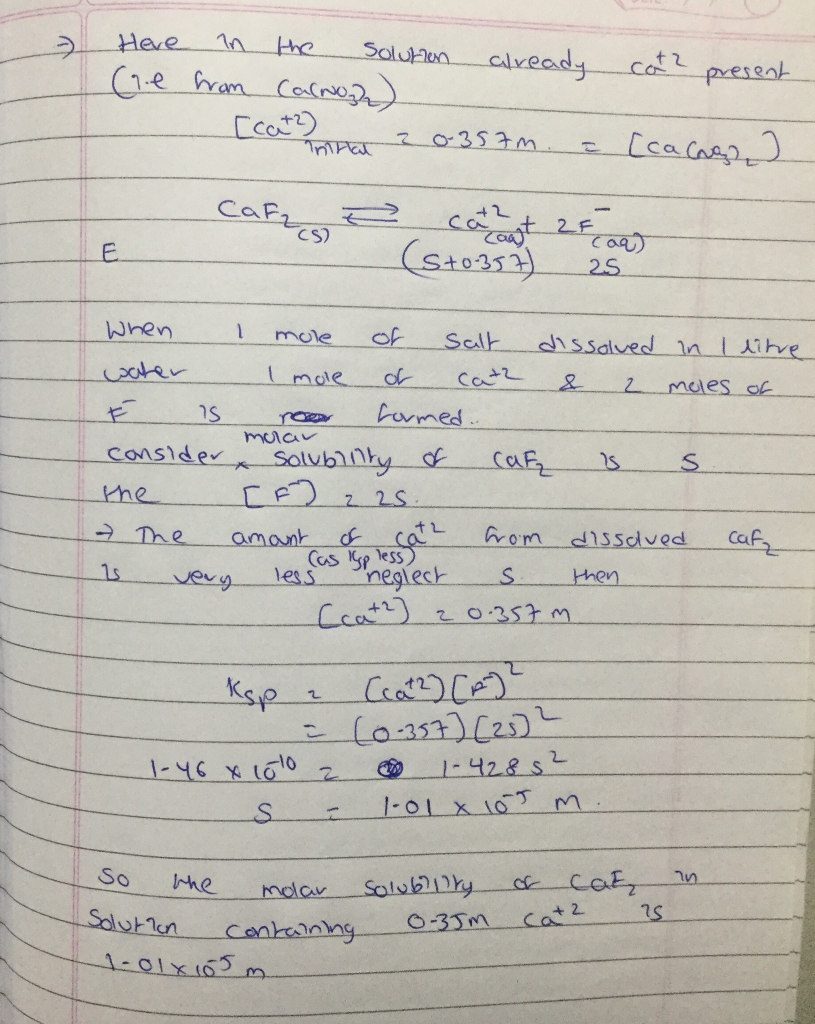Homework Help Question & Answers

Calculate the molar solubility of CaF2 in a solution containing 0.357 M of Ca(NO3)2. The Ksp valu...

Calculate the molar solubility of CaF2 in a solution containing 0.357 M of Ca(NO3)2. The Ksp value for CaF2 is 1.46×10−10.

Express the molar solubility to three significant figures and include the appropriate units.Add Answer of: Calculate the molar solubility of CaF2 in a solution containing 0.357 M of Ca(NO3)2. The Ksp valu...
More Homework Help Questions Additional questions in this topic.

• How many liters of a solution containing 22% sugar and how many liters of another solution containing 31% sugar must be mixed together to make 680 liters of solution containing 27% sugar

Need Online Homework Help?

Get FREE EXPERT Answers
WITHIN MINUTES
Related Questions